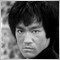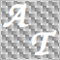• Overview
• Reviews (2)

# HVolatility

The HVolatility is the indicator that show the historical volatility generally known.

### Algorithm

At first, the logarithm between two prices is calculated. The CLOSE mode uses close prices at the arbitrary time and its previous. The HIGHLOW mode uses high and low prices at the arbitrary time.

```d[t] = ln(price[t] / price[t-1])
```

Then, the year rate is calculated by the followings. Its unit is second in this indicator.

`YearRate = sqrt(TradingDay * 24 * 60 * 60 / interval[t])`

That interval is calculated by the first time and end time in the period. The TradingDay is the trading day in any given year,

Finally, as the followings, the volatility is calculated.

`Volatility[t] = YearRate * StandardDeviationOf(d, period)`

The HVolatility uses the online algorithm for the variance calculation.

### Inputs

• TradingDay – trading days in any given year (default is 250)
• VolatilityMode1 – the way of calculation for the historical volatility1. CLOSE or HIGHLOW can be selected. (default is CLOSE)
• VolatilityPeriod1 – period of the historical volatility1 (default is 20)
• IndicatorType1 –the index style for volatility1. Line or Histogram can be selected. (default is Histogram)
• ShowVolatility2 – Show volatility2 (default is false).
• VolatilityMode2 – the way of calculation for the historical volatility2. CLOSE or HIGHLOW can be selected. (default is CLOSE)
• VolatilityPeriod2 – period of the historical volatility2 (default is 10)
• IndicatorType2 –the index style for volatility2. Line or Histogram can be selected. (default is Histogram)5432
2018.03.19 01:20

Does not represent volatility well21673
2016.08.31 08:08

User didn't leave any comment to the rating